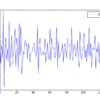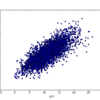# Archive | Time Series## How to Use Power Transforms for Time Series Forecast Data with Python

Data transforms are intended to remove noise and improve the signal in time series forecasting. It can be very difficult to select a good, or even best, transform for a given prediction problem. There are many transforms to choose from and each has a different mathematical intuition. In this tutorial, you will discover how to […]## How to Reframe Your Time Series Forecasting Problem

You do not have to model your time series forecast problem as-is. There are many ways to reframe your forecast problem that can both simplify the prediction problem and potentially expose more or different information to be modeled. A reframing can ultimately result in better and/or more robust forecasts. In this tutorial, you will discover […]## A Gentle Introduction to the Random Walk for Times Series Forecasting with Python

How do you know if your time series problem is predictable? This is a difficult question with time series forecasting. There is a tool called a random walk that can help you understand the predictability of your time series forecast problem. In this tutorial, you will discover the random walk and its properties in Python. […]## How to Grid Search ARIMA Model Hyperparameters with Python

The ARIMA model for time series analysis and forecasting can be tricky to configure. There are 3 parameters that require estimation by iterative trial and error from reviewing diagnostic plots and using 40-year-old heuristic rules. We can automate the process of evaluating a large number of hyperparameters for the ARIMA model by using a grid […]## A Gentle Introduction to the Box-Jenkins Method for Time Series Forecasting

The Autoregressive Integrated Moving Average Model, or ARIMA for short is a standard statistical model for time series forecast and analysis. Along with its development, the authors Box and Jenkins also suggest a process for identifying, estimating, and checking models for a specific time series dataset. This process is now referred to as the Box-Jenkins […]## How to Model Residual Errors to Correct Time Series Forecasts with Python

The residual errors from forecasts on a time series provide another source of information that we can model. Residual errors themselves form a time series that can have temporal structure. A simple autoregression model of this structure can be used to predict the forecast error, which in turn can be used to correct forecasts. This […]## How to Create an ARIMA Model for Time Series Forecasting in Python

A popular and widely used statistical method for time series forecasting is the ARIMA model. ARIMA is an acronym that stands for AutoRegressive Integrated Moving Average. It is a class of model that captures a suite of different standard temporal structures in time series data. In this tutorial, you will discover how to develop an […]## How to Visualize Time Series Residual Forecast Errors with Python

Forecast errors on time series regression problems are called residuals or residual errors. Careful exploration of residual errors on your time series prediction problem can tell you a lot about your forecast model and even suggest improvements. In this tutorial, you will discover how to visualize residual errors from time series forecasts. After completing this […]## Time Series Data Visualization with Python

6 Ways to Plot Your Time Series Data with Python Time series lends itself naturally to visualization. Line plots of observations over time are popular, but there is a suite of other plots that you can use to learn more about your problem. The more you learn about your data, the more likely you are […]## Autoregression Models for Time Series Forecasting With Python

Autoregression is a time series model that uses observations from previous time steps as input to a regression equation to predict the value at the next time step. It is a very simple idea that can result in accurate forecasts on a range of time series problems. In this tutorial, you will discover how to […]